# PSEB 5th Class Maths Solutions Chapter 3 HCF and LCM Ex 3.2

Punjab State Board PSEB 5th Class Maths Book Solutions Chapter 3 HCF and LCM Ex 3.2 Textbook Exercise Questions and Answers.

## PSEB Solutions for Class 5 Maths Chapter 3 HCF and LCM Ex 3.2

Question 1.
Write the Prime numbers from the following :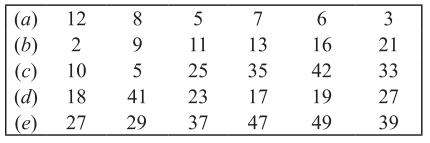Solution:
(a) 5, 7, 3
(b) 2, 11, 13
(c) 5
(d) 41, 23, 17, 19
(e) 41, 37, 47.

Question 2.
Write the composite numbers from the following :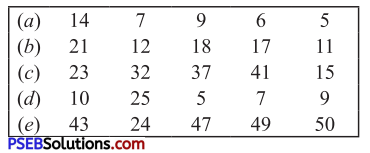Solution:
(a) 14, 9, 6
(b) 21, 12, 18
(c) 32, 15
(d) 10, 25, 9
(e) 24, 49, 50.Question 3.
Find HCF of following numbers using Prime factorisation.
(a) 18, 27
(b) 21, 63
(c) 80, 100
(d) 42, 98.
Solution: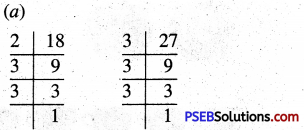Prime factorisation of 18 = 2 × 3 × 3
Prime factorisation of 27 = 3 × 3 × 3
Common Prime Factors = 3 and 3
∴ HCF of 18 and 27 = 3 × 3 = 9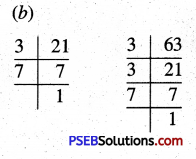Prime factorisation of 21 = 3 × 7
Prime factorisation of 63 = 3 × 3 × 7
Common Prime Factors = 3 and 7
∴ HCF of 21 and 63 = 3 × 7 = 21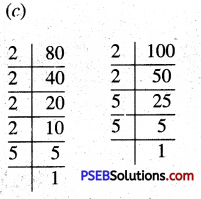Prime factorisation of 80 = 2 × 2 × 2 × 2 × 5
Prime factorisation of 100 = 2 × 2 × 5 × 5
Common Prime Factors = 2, 2 and 5
HCF of 80 and 100 = 2 × 2 × 5 = 20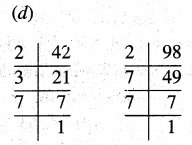Prime factorisation of 42 = 2 × 3 × 7
Prime factorisation of 98 = 2 × 7 × 7
Common Prime Factors = 2 and 7
HCF of 42 and 98 = 2 × 7 = 14Question 4.
Find HCF of following numbers using Prime factorisation :
(a) 30, 50, 70
(b) 24, 32, 40
(b) 36, 60, 72
(d) 25, 30, 35.
Solution: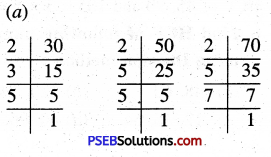Prime factorisation of 30 = 2 × 3 × 5
Prime factorisation of 50 = 2 × 5 × 5
Prime factorisation of 70 = 2 × 5 × 7
Common Prime Factors = 2 and 5
∴ HCF of 30, 50 and 70 = 2 × 5 = 10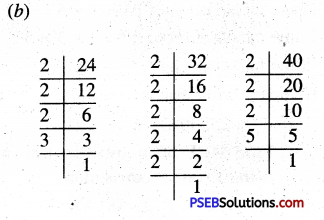Prime factorisation of 24 = 2 × 2 × 2 × 3
Prime factorisation of 32 = 2 × 2 × 2 × 2 × 2
Prime factorisation of 40 = 2 × 2 × 2 × 5
Common Prime Factors = 2, 2 and 2
∴ HCF of 24, 32 and 40 = 2 × 2 × 2 = 8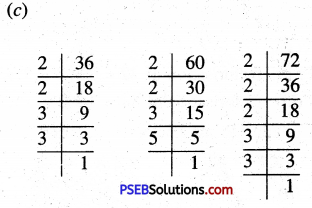Prime factorisation of 36 = 2 × 2 × 3 × 3
Prime factorisation of 60 = 2 × 2 × 3 × 5
Prime factorisation of 72 = 2 × 2 × 2 × 3 × 3
Common Prime Factors = 2, 2 and 3
∴ HCF of 36, 60 and 72 = 2 × 2 × 3 = 12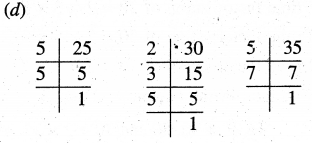Prime factorisation of 25 = 5 × 5
Prime factorisation of 30 = 2 × 3 × 5
Prime factorisation of 35 = 5 × 7
Common Prime Factor = 5
∴ HCF of 25, 30, 35 = 5

Question 5.
Find HCF of following numbers using Prime factorisation :
(a) 42, 84
(b) 45, 90
(c) 16, 64, 80
(d) 45, 90, 105.
Solution: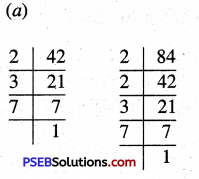Prime factorisation of 42 = 2 × 3 × 7
Prime factorisation of 84 = 2 × 2 × 3 × 7
Common Prime Factors = 2, 3 and 7
∴ HCF of 42, 84 = 2 × 3 × 7 = 42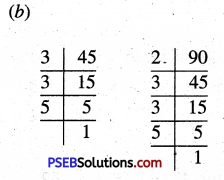Prime factorisation of 45 = 3 × 3 × 5
Prime factorisation of 90 = 2 × 3 × 3 × 5
Common Prime Factors = 3, 3 and 5
HCF of 45, 90 = 3 × 3 × 5 = 45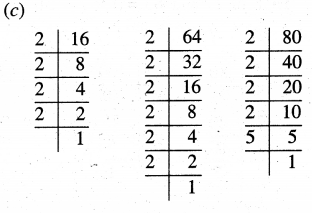Prime factorisation of 16 = 2 × 2 × 2 × 2
Prime factorisation of 64 = 2 × 2 × 2 × 2 × 2 × 2
Prime factorisation of 80 = 2 × 2 × 2 × 2 × 5
Common Prime Factors = 2, 2, 2 and 2
∴ HCF of 16, 64, 80 = 2 × 2 × 2 × 2 = 16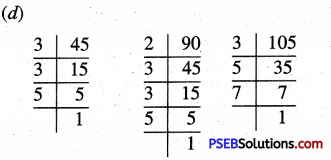Prime factorisation of 45 = 3 × 3 × 5
Prime factorisation of 90 = 2 × 3 × 3 × 5
Prime factorisation of 105 = 3 × 5 × 7
Common Prime Factors = 3 and 5
∴ HCF of 45, 90 and 105 = 3 × 5 = 15

Question 6.
Find HCF of following numbers using Division method :
(a) 48, 60
(b) 120, 140
(c) 12, 18, 64
(d) 60, 96, 128
Solution:
(a)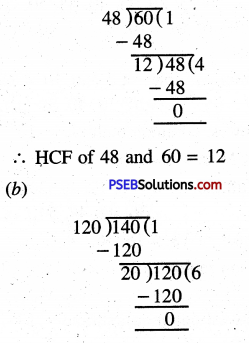∴ HCF of 120 and 140 = 20
(c) First find HCF of any two numbers say 12 and 18.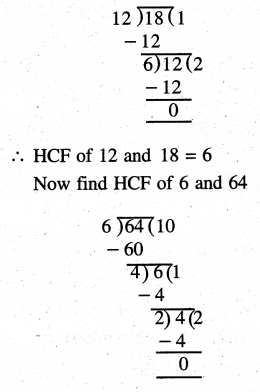∴ HCF of 12, 18 and 64 = 2

(d) First find HCF of any two numbers say 60 and 96.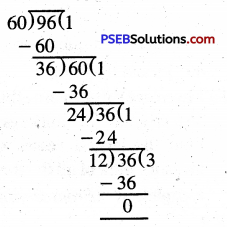∴ HCF of 60 and 96 = 12
Now find HCF of 12 and 128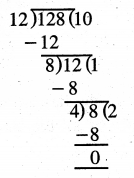∴ HCF of 60, 96, 128 = 4Question 7.
Find the largest number which divides 60, 75 and 90 without leaving any remainder.
Solution:
The largest number which divides 60, 75 and 90 without leaving any remainder = HCF of 60, 75 and 90
First find HCF of any two numbers say 60 and 75.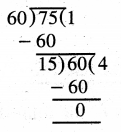∴ HCF of 60 and 75 = 15
Now, find HCF of 15 and 90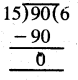The greatest number which divides 60, 75 and 90 = 15

Question 8.
There are three drums that contain 36l, 45l, and 72l milk respectively. Find the largest vessel which can measure milk of all the three drums completely.
Solution: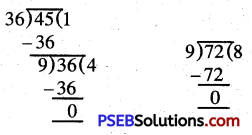The capacity of the largest vessel that can measure milk of all the three drums completely = 9 l.